Request a Tool

Peclet Number Calculator

Peclet Number is a dimensionless number that deals in the study of transport phenomena in fluid flows.

Velocity
0

Formula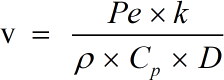• v = Velocity
• ρ = Density
• Cp = Heat Capacity
• D = Characteristic Length
• k = Thermal Conductivity
• Pe = Peclet Number

Defination / Uses

The concept of velocity for an object is the rate at which the object's position changes in relation to a frame of reference and time. It's a vector quantity, which means we need both magnitude (speed) and direction (orientation) to define velocity. Its SI unit is meter per second (m/s). When the amount or direction of a body's velocity changes, the body is said to be accelerating.

Density
0

Formula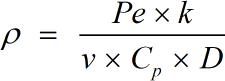• ρ = Density
• Cp = Heat Capacity
• D = Characteristic Length
• k = Thermal Conductivity
• Pe = Peclet Number
• v = Velocity

Defination / Uses

The mass of a material substance per unit volume is called density. The density of a substance is usually measured in grammes per cm³.

Heat Capacity
0

Formula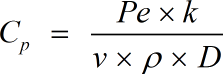• Cp = Heat Capacity
• D = Characteristic Length
• k = Thermal Conductivity
• Pe = Peclet Number
• v = Velocity
• ρ = Density

Defination / Uses

The amount of heat energy that must be absorbed to raise the temperature of one gramme of a substance by one degree Celsius is known as heat capacity.

Output

Characteristic Length
0

Formula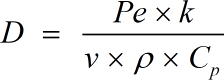• D = Characteristic Length
• k = Thermal Conductivity
• Pe = Peclet Number
• v = Velocity
• ρ = Density
• Cp = Heat Capacity

Defination / Uses

Characteristic length is a crucial dimension in physics that specifies the scale of a physical system. Characteristic length is defined in computational mechanics to force localization of a stress softening constitutive equation. An integration point is referred to the length.

Output

Thermal Conductivity
0

Formula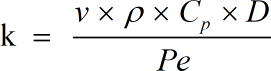• k = Thermal Conductivity
• Pe = Peclet Number
• v = Velocity
• ρ = Density
• Cp = Heat Capacity
• D = Characteristic Length

Defination / Uses

The Peclet Number is the ratio of the rate of advection of a physical quantity by a flow to the rate of diffusion of the same quantity driven by an appropriate gradient. It takes into account heat capacity, density, fluid velocity, and other physical factors. The sign "Pe" stands for Peclet Number.

To put it another way, Peclet Number is a dimensionless group that is used to scale up chemical reaction similitude from pilot-plant data to commercial-sized units.

Peclet Number
0

Formula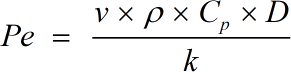• Pe = Peclet Number
• v = Velocity
• ρ = Density
• Cp = Heat Capacity
• D = Characteristic Length
• k = Thermal Conductivity

Defination / Uses

The Peclet Number is the ratio of the rate of advection of a physical quantity by a flow to the rate of diffusion of the same quantity driven by an appropriate gradient. It takes into account heat capacity, density, fluid velocity, and other physical factors. The sign "Pe" stands for Peclet Number.

To put it another way, Peclet Number is a dimensionless group that is used to scale up chemical reaction similitude from pilot-plant data to commercial-sized units.

How to use peclet number calcultor?

There are only two basic steps.

• First select the option from dropdown. What you need to calculate.
• Then add the other values in their corresponding fields.